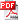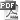This course explores advances in algorithm design, algorithm analysis and data structures. The primary focus is on advanced data structures. Topics include advanced trees structures, disjoint sets, heaps, algorithm design techniques, data structures for strings and linear programming algorithms. Finally, we discuss NP-completeness.

Competitive ProgrammingAsytmptotic Analysis for recursive functionsHacks on Bitwise ProgrammingDisjoint SetsAlgorithm design techniquesGreedy algorithmsDivide and ConquerDynamic ProgrammingGraph - Graph TraversalGraph - Minimum Spanning TreeGraph - Single-Source Shortest PathsGraph - All-Pairs Shortest PathsGraph - Network FlowGraph - Special GraphsGraph - Graph MatchingMathematicsString ProcessingComputational Geometry